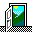## Programming the HP 40gs Graphing Calculator

Listings of programs I have written some years ago for the HP 40gs calculator:### Helmert Transformation (2D)

A small program to transform coordinates from a source system (`y`, `x`) to a target system (`Y`, `X`).

Input:

• identical points (`y`, `x`) in source system in `C1`, `C2`
• identical points (`Y`, `X`) in target system in `C3`, `C4`
(number of identical points n >= 2)
• points to transform (`y`, `x`) in source system in `C5`, `C6`

Output:

• parameters `o`, `a`
• scale `m`
• variances `WY`, `WX`
• standard deviation `s`
• transformed points (`Y`, `X`) in target system in `C7`, `C8`

#### Source Code

``````ERASE:
DISP 1; "Helmert Transformation":

SELECT Statistics:

SIZE(C1) ▶ N:
SIZE(C5) ▶ P:

MAKELIST(0, A, 1, N, 1) ▶ L1:
L1 ▶ L2:
L1 ▶ L3:
L1 ▶ L4:
L1 ▶ L5:
L1 ▶ L6:

MAKELIST(0, A, 1, P, 1) ▶ C7:
C7 ▶ C8:

ΣLIST(C1) / N ▶ C:
ΣLIST(C2) / N ▶ D:
ΣLIST(C3) / N ▶ E:
ΣLIST(C4) / N ▶ F:

FOR I = 1 TO N;
C1(I) - C ▶ L1(I):
C2(I) - D ▶ L2(I):
C3(I) - E ▶ L3(I):
C4(I) - F ▶ L4(I):
END

FOR I = 1 TO N;
L2(I) * L3(I) - L1(I) * L4(I) ▶ L5(I):
L2(I)² + L1(I)² ▶ L6(I):
END:

ΣLIST(L5) / ΣLIST(L6) ▶ O:
DISP 1; "o=" O:

FOR I = 1 TO N;
L2(I) * L4(I) + L1(I) * L3(I) ▶ L5(I):
END:

ΣLIST(L5) / ΣLIST(L6) ▶ A:
DISP 2; "a=" A:

E - A * C - O * D ▶ Y:
F - A * D + O * C ▶ X:

√(A² + O²) ▶ M:
DISP 3; "m=" M;

0 ▶ V:
0 ▶ W:

FOR I = 1 TO N;
-Y - A * C1(I) - O * C2(I) + C3(I) ▶ L5(I):
-X - A * C2(I) + O * C1(I) + C4(I) ▶ L6(I):
V + L5(I)² ▶ V:
W + L6(I)² ▶ W:
END:

DISP 4; "WY=" ΣLIST(L5) " WX=" ΣLIST(L6):

√((V + W) / (2 * N - 4)) ▶ S:
DISP 5; "s=" S * 1000 "mm":

DISP 7; "Writing to C7/C8...":

FOR I = 1 TO P;
Y + A * C5(I) + O * C6(I) ▶ C7(I):
X + A * C6(I) - O * C5(I) ▶ C8(I):
END:

DISP 7; "Done.":

CLRVAR L1: CLRVAR L2:
CLRVAR L3: CLRVAR L4:
CLRVAR L5: CLRVAR L6:

FREEZE:``````

#### References

• Franz Josef Gruber, Rainer Jockel: Formelsammlung für das Vermessungswesen (Teubner, 2007)### Normalised Euler Spiral

A tiny program to calculate the arc point coordinates of a normalised Euler spiral with respect to the arc length `L`.

Input:

• arc length `L`

Output:

• coordinates of arc point

#### Source Code

``````ERASE:

DISP 1; "Normalised Euler spiral":

0 ▶ J:
-1 ▶ K:

PROMPT L:

L ▶ A:
A ▶ X:
0 ▶ Y:

DO
J + 2 ▶ J:
-K ▶ K:
A * L * L * (J - 1) / (J * (J + 1)) ▶ A:

IF K < 0 THEN
-A ▶ A:
X + A ▶ X:
ELSE
Y + A ▶ Y:
END:
UNTIL
ABS(A) < .5 * 10^-7
END:

ERASE:

DISP 1; "Arc point":
DISP 2; "X: " X:
DISP 3; "Y: " Y:

CLRVAR J: CLRVAR K:
CLRVAR L: CLRVAR A:
CLRVAR X: CLRVAR Y:

FREEZE:``````